﻿

### On a class of rational matrices and interpolating polynomials related to the discrete Laplace operator

#### Abstract

Let$\dlap$ be the discrete Laplace operator acting on functions(or rational matrices)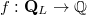$f:\mathbf{Q}_L\rightarrow\mathbb{Q}$,where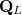$\mathbf{Q}_L$ is the two dimensional lattice of size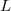$L$embedded in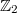$\mathbb{Z}_2$. Consider a rational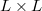$L\times L$ matrix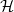$\mathcal{H}$, whose inner entries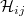$\mathcal{H}_{ij}$ satisfy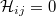$\dlap\mathcal{H}_{ij}=0$. The matrix$\mathcal{H}$ is thus theclassical finite difference five-points approximation of theLaplace operator in two variables. We give a constructive proofthat$\mathcal{H}$ is the restriction to$\mathbf{Q}_L$ of adiscrete harmonic polynomial in two variables for any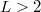$L>2$. Thisresult proves a conjecture formulated in the context ofdeterministic fixed-energy sandpile models in statisticalmechanics.

DOI Code: 10.1285/i15900932v28n2p1

Keywords: rational matrices; discrete Laplacian; discrete harmonic polynomials; sandpile

Classification: 11C99 (Polynomials and matrices)

Full Text: PDF